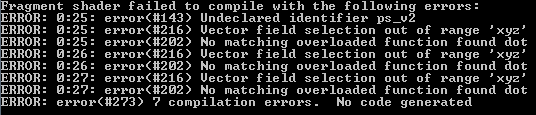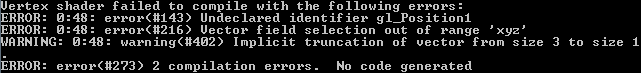# Shader code dropping normal when translating to GLSL - Monogame bug?

I’m trying to make a shader that is specular enabled, but I can’t seem to get the normal passed in to my fragment shader.

``````struct VertexData
{
float4 position : POSITION0;
float4 color : COLOR0;
float4 specularColor : COLOR1;
float3 normal : NORMAL0;
};

struct VertexToPixel
{
float4 position : POSITION0;
float4 litColor : COLOR0;
float4 specularColor : COLOR1;
float3 normal : NORMAL0;  //It refuses to recognize this
};

{
VertexToPixel output;

float4 worldPosition = mul(input.position, World);
float4 viewPosition = mul(worldPosition, View);
output.position = mul(viewPosition, Projection);

float4 lightColor = ambientColor * ambientColor.a;

//normally we'd need to switch the normal to be in world-space
//so we cool.
//as long as we don't do any world space rotation.
float diffuseIntensity = dot(input.normal, -sunDir) * sunColor.a;
if (diffuseIntensity > 0)
lightColor = lightColor + sunColor * diffuseIntensity;

output.litColor = float4(0, 0, 0, 0);

output.litColor.rgb = input.color.rgb * lightColor.rgb;
output.litColor.a = input.color.a;

output.litColor = saturate(output.litColor);

output.specularColor = input.specularColor;
output.normal = input.normal;

return output;
}

{
float3 eyePosition = float3(0, 0, 1.0);

float3 worldNormal = mul(float4(input.normal, 0), World).xyz;
float3 reflection = normalize(sunDir - (2 * worldNormal * dot(sunDir, worldNormal)));
reflection = mul(float4(reflection, 0), View).xyz;

float3 specularResult = input.specularColor.rgb * sunColor.rgb * pow(dot(reflection, eyePosition), input.specularColor.a);

//This next is an experimental function to
float alphaFactor = max(max(specularResult.r, specularResult.g), specularResult.b);

float3 premultSurfaceColor = input.litColor.rgb * input.litColor.a;

float4 finalColor = float4(0, 0, 0, 0);
finalColor.rgb = specularResult.rgb + (premultSurfaceColor.rgb * (1 - alphaFactor));
finalColor.a = alphaFactor + input.litColor.a * (1 - alphaFactor);

return finalColor;
}
``````

But it gives me this error:The main error is the first, the others are just caused by that variable being missing.

This is the GLSL code my fragment shader (and header) got translated to:

``````    uniform vec4 ps_uniforms_vec4;
const vec4 ps_c8 = vec4(1.0, 0.0, 0.0, 0.0);
vec4 ps_r0;
vec4 ps_r1;
vec4 ps_r2;
#define ps_c0 ps_uniforms_vec4
#define ps_c1 ps_uniforms_vec4
#define ps_c2 ps_uniforms_vec4
#define ps_c5 ps_uniforms_vec4
#define ps_c6 ps_uniforms_vec4
#define ps_c7 ps_uniforms_vec4
varying vec4 vFrontColor;
#define ps_v0 vFrontColor
#define ps_oC0 gl_FragColor
varying vec4 vFrontSecondaryColor;
#define ps_v1 vFrontSecondaryColor

void main()
{
ps_r0.x = dot(ps_v2.xyz, ps_c0.xyz);
ps_r0.y = dot(ps_v2.xyz, ps_c1.xyz);
ps_r0.z = dot(ps_v2.xyz, ps_c2.xyz);
ps_r1.xyz = ps_r0.xyz + ps_r0.xyz;
ps_r0.x = dot(ps_c6.xyz, ps_r0.xyz);
ps_r0.xyz = (ps_r1.xyz * -ps_r0.xxx) + ps_c6.xyz;
ps_r1.xyz = normalize(ps_r0.xyz);
ps_r0.x = dot(ps_r1.xyz, ps_c5.xyz);
ps_r1.x = pow(abs(ps_r0.x), ps_v1.w);
ps_r0.xyz = ps_c7.xyz * ps_v1.xyz;
ps_r0.xyz = ps_r1.xxx * ps_r0.xyz;
ps_r1.x = max(ps_r0.x, ps_r0.y);
ps_r2.x = max(ps_r1.x, ps_r0.z);
ps_r0.w = -ps_r2.x + ps_c8.x;
ps_oC0.w = (ps_v0.w * ps_r0.w) + ps_r2.x;
ps_r1.xyz = ps_v0.www * ps_v0.xyz;
ps_oC0.xyz = (ps_r1.xyz * ps_r0.www) + ps_r0.xyz;
}
``````

Did I do something wrong in the syntax or is the code translator just arbitrarily dropping variables?

Hi,

I don’t know much of shaders, and less of GL shaders, but in the past I had lots of problems because the shader optimized the unused variables. If you’re not setting the normal in the vertex shader it’ll probably optimize it out.

Jup, “the effect compiler is really aggressive” and other useful stuff on writing effects can be found here

Yeah, but I am setting the normal…

Adding the vertex shader code to OP since at least it eliminates some possible causes.

Did you try this shader with DX? If that works the problem is probably with MojoShader. Else you could try debugging 2MGFX, but I imagine that’s not easy.

Well, I’ve done that now, and it works properly in DX (well, after changing to the higher shader versions, and my math is wrong, but the point is it passes the normal correctly.)

Huh. So after using the DirectX test version to fix the incorrect math, the OpenGl version is now giving me a different error. Probably related but not sure. I’ll just post the new stuff here…

Original HLSL code:

``````float4x4 World;
float4x4 View;
float4x4 Projection;

float4 ambientColor = float4(1, 1, 1, 1);

float3 sunDir = float3(0.1, -1, 0.1);
float4 sunColor = float4(1, 1, 0.8, 1);

float3 eyePosition = float3(0, 0, -1.0);

struct VertexData
{
float4 position : POSITION0;
float4 color : COLOR0;
float4 specularColor : COLOR1;
float3 normal : NORMAL0;
};

struct VertexToPixel
{
float4 position : POSITION0;
float4 litColor : COLOR0;
float4 specularColor : COLOR1;
float3 normal : NORMAL0;
float3 worldPosition : POSITION1; //now it's this one that it doesn't recognize
//can I workaround by adding another definition that I don't use?
};

{
VertexToPixel output;

float4 worldPosition = mul(input.position, World);
float4 viewPosition = mul(worldPosition, View);
output.position = mul(viewPosition, Projection);
output.worldPosition = worldPosition.xyz;

float4 lightColor = ambientColor * ambientColor.a;

float diffuseIntensity = dot(input.normal, -sunDir) * sunColor.a;
if (diffuseIntensity > 0)
lightColor = lightColor + sunColor * diffuseIntensity;

output.litColor = float4(0, 0, 0, 0);

output.litColor.rgb = input.color.rgb * lightColor.rgb;
output.litColor.a = input.color.a;

output.litColor = saturate(output.litColor);

output.specularColor = input.specularColor;
output.normal = input.normal;

return output;
}

{
float3 worldNormal = normalize(mul(float4(input.normal, 0), World).xyz);
float3 normSunDir = normalize(sunDir);
float3 reflection = normalize(sunDir - (2 * worldNormal * dot(normSunDir, worldNormal)));

float3 eyeVector = eyePosition - input.worldPosition;

float angle = dot(reflection, normalize(eyeVector));

float3 premultSurfaceColor = input.litColor.rgb * input.litColor.a;

if (angle < 0)
return float4(premultSurfaceColor, input.litColor.a);

float specularPower = input.specularColor.a * 255;

float3 specularResult = input.specularColor.rgb * sunColor.rgb * pow(angle, specularPower);

float alphaFactor = max(max(specularResult.r, specularResult.g), specularResult.b);

float4 finalColor = float4(0, 0, 0, 0);
finalColor.rgb = specularResult.rgb + (premultSurfaceColor.rgb * (1 - alphaFactor));
finalColor.a = alphaFactor + input.litColor.a * (1 - alphaFactor);

return finalColor;
}

{
pass Pass1
{
}
}``````

Which then gets translated to this GLSL code: (omitting the sections with all the [NUL]s and whatnot)

``````#ifdef GL_ES
precision mediump float;
precision mediump int;
#endif

uniform vec4 ps_uniforms_vec4;
const vec4 ps_c6 = vec4(255.0, 1.0, 0.0, 0.0);
vec4 ps_r0;
vec4 ps_r1;
vec4 ps_r2;
vec4 ps_r3;
#define ps_c0 ps_uniforms_vec4
#define ps_c1 ps_uniforms_vec4
#define ps_c2 ps_uniforms_vec4
#define ps_c3 ps_uniforms_vec4
#define ps_c4 ps_uniforms_vec4
#define ps_c5 ps_uniforms_vec4
varying vec4 vFrontColor;
#define ps_v0 vFrontColor
#define ps_oC0 gl_FragColor
varying vec4 vFrontSecondaryColor;
#define ps_v1 vFrontSecondaryColor

void main()
{
ps_r0.x = dot(ps_v2.xyz, ps_c0.xyz);
ps_r0.y = dot(ps_v2.xyz, ps_c1.xyz);
ps_r0.z = dot(ps_v2.xyz, ps_c2.xyz);
ps_r1.xyz = normalize(ps_r0.xyz);
ps_r0.xyz = normalize(ps_c3.xyz);
ps_r0.x = dot(ps_r0.xyz, ps_r1.xyz);
ps_r0.yzw = ps_r1.xyz + ps_r1.xyz;
ps_r0.xyz = (ps_r0.yzw * -ps_r0.xxx) + ps_c3.xyz;
ps_r1.xyz = normalize(ps_r0.xyz);
ps_r0.xyz = ps_c5.xyz + -ps_v3.xyz;
ps_r2.xyz = normalize(ps_r0.xyz);
ps_r0.x = dot(ps_r1.xyz, ps_r2.xyz);
ps_r0.y = ps_c6.x * ps_v1.w;
ps_r1.x = pow(abs(ps_r0.x), ps_r0.y);
ps_r0.yzw = ps_c4.xyz * ps_v1.xyz;
ps_r0.yzw = ps_r1.xxx * ps_r0.yzw;
ps_r1.x = max(ps_r0.y, ps_r0.z);
ps_r2.x = max(ps_r1.x, ps_r0.w);
ps_r1.x = -ps_r2.x + ps_c6.y;
ps_r3.w = mix(ps_r2.x, ps_c6.y, ps_v0.w);
ps_r2.xyz = ps_v0.www * ps_v0.xyz;
ps_r3.xyz = (ps_r2.xyz * ps_r1.xxx) + ps_r0.yzw;
ps_r2.w = ps_v0.w;
ps_oC0 = ((ps_r0.x >= 0.0) ? ps_r3 : ps_r2);
}

#ifdef GL_ES
precision highp float;
precision mediump int;
#endif

uniform vec4 vs_uniforms_vec4;
uniform vec4 posFixup;
const vec4 vs_c15 = vec4(0.0, 0.0, 0.0, 0.0);
vec4 vs_r0;
vec4 vs_r1;
#define vs_c0 vs_uniforms_vec4
#define vs_c1 vs_uniforms_vec4
#define vs_c2 vs_uniforms_vec4
#define vs_c3 vs_uniforms_vec4
#define vs_c4 vs_uniforms_vec4
#define vs_c5 vs_uniforms_vec4
#define vs_c6 vs_uniforms_vec4
#define vs_c7 vs_uniforms_vec4
#define vs_c8 vs_uniforms_vec4
#define vs_c9 vs_uniforms_vec4
#define vs_c10 vs_uniforms_vec4
#define vs_c11 vs_uniforms_vec4
#define vs_c12 vs_uniforms_vec4
#define vs_c13 vs_uniforms_vec4
#define vs_c14 vs_uniforms_vec4
attribute vec4 vs_v0;
#define vs_o0 gl_Position
attribute vec4 vs_v1;
varying vec4 vFrontColor;
#define vs_o1 vFrontColor
attribute vec4 vs_v2;
varying vec4 vFrontSecondaryColor;
#define vs_o2 vFrontSecondaryColor
attribute vec4 vs_v3;
vec4 vs_o3;
#define vs_o4 gl_Position1

void main()
{
vs_r0.w = dot(vs_v0, vs_c3);
vs_r0.x = dot(vs_v0, vs_c0);
vs_r0.y = dot(vs_v0, vs_c1);
vs_r0.z = dot(vs_v0, vs_c2);
vs_r1.x = dot(vs_r0, vs_c4);
vs_r1.y = dot(vs_r0, vs_c5);
vs_r1.z = dot(vs_r0, vs_c6);
vs_r1.w = dot(vs_r0, vs_c7);
vs_o4.xyz = vs_r0.xyz;
vs_o0.x = dot(vs_r1, vs_c8);
vs_o0.y = dot(vs_r1, vs_c9);
vs_o0.z = dot(vs_r1, vs_c10);
vs_o0.w = dot(vs_r1, vs_c11);
vs_r0.x = dot(vs_v3.xyz, -vs_c13.xyz);
vs_r0.x = vs_r0.x * vs_c14.w;
vs_r0.y = float(vs_c15.x < vs_r0.x);
vs_r0.xzw = vs_r0.xxx * vs_c14.xyz;
vs_r0.xyz = vs_r0.xzw * vs_r0.yyy;
vs_r0.xyz = (vs_c12.xyz * vs_c12.www) + vs_r0.xyz;
vs_o1.xyz = clamp(vs_r0.xyz * vs_v1.xyz, vec3(0.0), vec3(1.0));
vs_o1.w = clamp(vs_v1.w, 0.0, 1.0);
vs_o2 = vs_v2;
vs_o3.xyz = vs_v3.xyz;
gl_Position.y = gl_Position.y * posFixup.y;
gl_Position.xy += posFixup.zw * gl_Position.ww;
gl_Position.z = gl_Position.z * 2.0 - gl_Position.w;
}``````

Which then produces these errors:For the record, line 48 is this one:

``    ps_r2.w = ps_v0.w;``